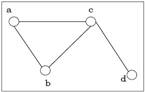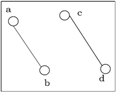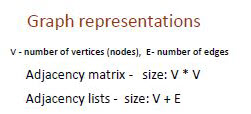# Algorithms

Classified in Language

Written at onEnglish with a size of 41.41 KB.

 Tweet

### Connected and Disconnected Graph: A graph is connected if any two vertices of the graph are connected by a path and a graph is disconnected if at least two vertices of the graph are not connected by a path. If a graph G is unconnected, then every maximal connected subgraph of G is called a connected component of the graph G.

Dense graph is a graph in which the number of edges is close to the maximal number of edges.Sparse graph is a graph in which the number of edges is close to the minimal number of edges. Sparse graph can be a disconnected graph.

• Informally, a graph with relatively few edges is sparse, and a graph with many edges is dense.
• Sparse graphs are sparsely connected (eg: Trees). Usually the number of edges is in O(n) where n is the number of vertices. Therefore adjacency lists are preferred since they require constant space for every edge.
• Dense graphs are densely connected. Here number of edges is usually O(n^2). Therefore adjacency matrix is preferred.
• To give a comparison, let us assume graph has 1000 vertices. Irrespective of whether the graph is dense or sparse, adjacency matrix requires 1000^2 = 1,000,000 values to be stored.
• If the graph is minimally connected (i.E. It is a tree), the adjacency list requires storing 2,997 values. If the graph is fully connected it requires storing 3,000,000 values.• ## The edges of a tree are known as branches. Elements of trees are called their nodes. The nodes without child nodes are called leaf nodes.

• A tree with ‘n’ vertices has ‘n-1’ edges. If it has one more edge extra than ‘n-1’, then the extra edge should obviously has to pair up with two vertices which leads to form a cycle. Then, it becomes a cyclic graph which is a violation for the tree graph.